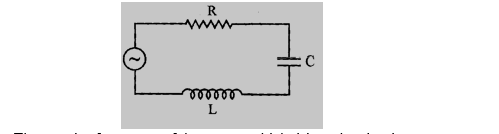# NEET Physics Alternating Current Questions Solved

The figure shows a series LCR circuit with L = 5.0 H, C = 80 µF, R = 40 Ω connected to a variable frequency 240 V source. Calculate(i) The angular frequency of the source which drives the circuit at resonance.

(ii) The current at the resonating frequency.

(iii)The rms potential drop across the capacitor at resonance.                                    3mark